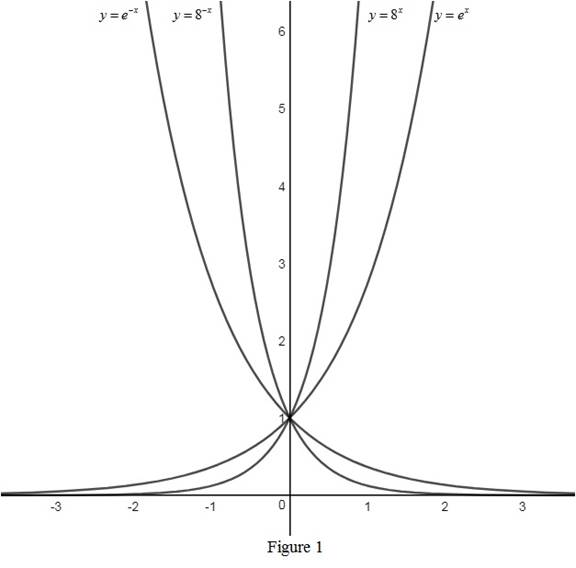# The graphs of the function y = e x , y = e − x , y = 8 x and y = 8 − x on the same axes and interpret how these graphs are related.### Single Variable Calculus: Concepts...

4th Edition
James Stewart
Publisher: Cengage Learning
ISBN: 9781337687805### Single Variable Calculus: Concepts...

4th Edition
James Stewart
Publisher: Cengage Learning
ISBN: 9781337687805

#### Solutions

Chapter 1.5, Problem 8E
To determine

## To sketch: The graphs of the function y=ex,y=e−x,y=8x and y=8−x on the same axes and interpret how these graphs are related.

Expert Solution

### Explanation of Solution

The rough graphs of the functions y=ex,y=ex,y=8x and y=8x are drawn and shown below in Figure 1.From Figure 1, it is observed that the graphs of the functions are passing through the common point (0, 1) as the value of e0 is always 1.

Also, notice that the functions y=ex and y=8x approaches as x tending to and approaches 0 as x tending to as they have the positive exponents. Therefore, the graphs are monotonically increasing.

Similarly, the functions y=ex and y=8x approaches 0 as x tending to and approaches as x tending to as they have the positive exponents. Therefore, the graphs are monotonically decreasing.

### Have a homework question?

Subscribe to bartleby learn! Ask subject matter experts 30 homework questions each month. Plus, you’ll have access to millions of step-by-step textbook answers!